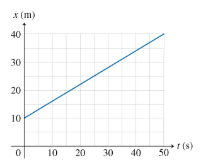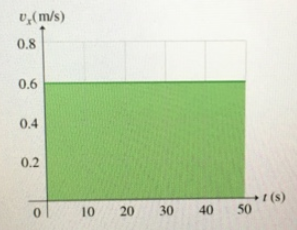# Problem: Figure 1:Figure 2:Shown in the figure is the v vs. t curve selected in the previous part. What is the area A of the shaded region under the curve?

###### FREE Expert Solution

The area under a velocity-time graph is the displacement.

The shape of the shaded region is rectangular.

84% (391 ratings)###### Problem Details

Figure 1:Figure 2:Shown in the figure is the v vs. t curve selected in the previous part. What is the area A of the shaded region under the curve?